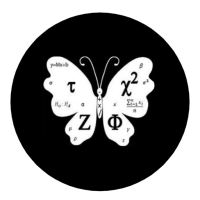## Welcome to Moore Statistics Consulting LLCThe Pearson’s Correlation Coefficient is typically used to evaluate two measurements to each other. The objective is to determine if there is a relationship between the two variables. You want to know if the two measures are moving in the same direction. The variables could be increasing at the same time; one could be decreasing while the other is increasing; or no relationship at all. And the association between the two variables is evaluated on a scale from -1 to 1. The close you are to either -1 to 1 , the stronger the relationship. A negative correlation represents one variable decreasing while the other is increasing over time. A positive correlation represents both variables increasing over time. Are you dizzy yet? Because I am…Okay here is the deal. If you report the Pearson’s Correlation Coefficient you need to include confidence intervals. Confidence intervals indicate whether or not zero is in the interval. If zero is in the interval then your Pearson’s correlation is not statistically significant.You should generate a p-value from your statistical program. I would report the p-value as well.

So the moral of this story is make sure you report more than your Pearson’s Correlation Coefficient and your test statistic needs to be statistically significant. One last thing, if you are trying to evaluate whether or not two measures are in agreement that is not the Pearson’s Correlation. There are specific tests to evaluate agreement which speaks more towards the magnitude of your values.The real last thing, there are correlation statistics specifically for variables that are not continuous. So you are not limited to Pearson’s Correlation Coefficient.

I hope you enjoyed this short blog, there are more to follow-Amy